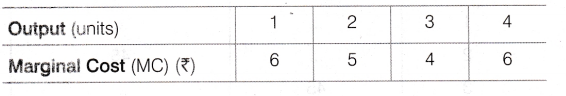# Calculate Total Variable Cost

Calculate Total Variable Cost and Total Cost from the following cost schedule of a firm whose Fixed
Costs are Rs.10